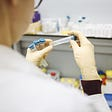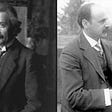# A Neat Use of Fibonacci Numbers

I love Fibonacci numbers. I first learned about them in grade school and ever since then I’ve enjoyed playing with the sequence. I’ve drawn art based on the Fibonacci series, been fascinated by the fractal pattern of the Fibonacci Spiral, and designed and knit a scarf for a good friend that incorporated a Fibonacci sequence in the pattern.

Recently I learned that Fibonacci numbers can be used to convert kilometers to miles and back. How cool is that???

## What are Fibonacci numbers

The basic concept behind the Fibonacci sequence is absurdly simple. Typically starting with 0 and 1, you just add the two numbers to get the next one, then shift and repeat.

This results in the following sequence:

0, 1, 1, 2, 3, 5, 8, 13, 21, 34, 55, 89, 144, …

## Origins

Apparently the first known description came from India, sometime between the 7th and 9th century A.D. It wasn’t until the 13th century that Fibonacci introduced the Western world to the concept.

Fibonacci numbers are extremely useful. Applications include not just the above mentioned fractal spiral pattern but also computational search techniques, a Fibonacci heap structure for data, and Fibonacci cubes that interconnect distributed systems.

Biological applications are numerous as we find Fibonacci sequences in the the leaf patterns of artichokes, in pine cone bracts, the branching patterns of trees, and many other instances that appear in nature.

Fibonacci numbers are also related to both Lucas sequences and the golden ratio.

## How to convert miles to kilometers and back using Fibonacci numbers

A friend of mine and I were talking about the metric system when he mentioned this nifty trick to me.

Say you want to convert

I recently learned an interesting fact about Fibonacci numbers while watching a random number theory video on YouTube. Fibonacci numbers can be used to approximately convert from miles to kilometers and back.

Here is how.

Let’s say you want to convert 3 miles to kilometers. The next number in the Fibonacci series after 3 is 5. And guess what, 3 miles is 4.83 kilometers, which is very close to 5. The conversion approximates the Fibonacci series.

For 5 miles — the next Fibonacci number is 8 and 5 miles is 8.03 kilometers. Want to go from km to miles? Easy — 8 km is about 5 miles.

How about larger numbers? No problems. 34 km converts to 21 miles and vice versa.

The caveat of course is that these are all approximations. In the example above, 21 miles is accurately converted to 33.79 km. So while this method yields a quick and easy back-of-the-envelope conversion, if you need precision, you’ll need to crunch the numbers.

## Further ramifications

What about if you want to convert a non-Fibonacci number?

Simply break it down into a sum of Fibonacci numbers and proceed as shown.

For example, 10 miles can be expressed as 8 + 2. This converts to 13 + 3 or 16 km. The actual number? 16.0934.

How about 100 miles? That can be expressed as 89 + 8 + 3. When you shift to the next numbers in the series you get 144 + 13 + 5. This adds up to 162 km, only 1% off from the actual conversion of 160.93 km.

Want to convert 160 km back to miles? Just shift the series in the opposite direction, going with the prior number in the sequence instead of the next. Are we having fun yet?

## Why does this work so well?

It comes down to the golden ratio, which is approximately 1.618. There are 1.609 kilometers per mile. That is within 0.5% of the golden ratio. Since the Fibonacci sequence progressively approaches the golden ratio, it more exactly approximates the ratio as the numbers get larger.

These key facts help to make the Fibonacci sequence a really useful tool for approximating metric to imperial conversions and vice versa.

--

--

--

## More from Shefali O'Hara

Cancer survivor, artist, writer, engineer. Grew up in NYC, living in Austin. Love animals, books, hikes, art, travel, D&D and fireworks.

Love podcasts or audiobooks? Learn on the go with our new app.

## Fibonacci Numbers and Generating Functions## Conditional Probability: Why Tested Positive Doesn't Necessarily Mean You Got Corona Virus## Let’s Talk about Maths and Gambling!## Karl Schwarzschild’s Letter to Albert Einstein## Reasoning under uncertainty with a near-term quantum computer## Shefali O'Hara

Cancer survivor, artist, writer, engineer. Grew up in NYC, living in Austin. Love animals, books, hikes, art, travel, D&D and fireworks.

## How to have glowing skin## The Food Lover’s Search For Bold Spices and Answers to Questions About Authenticity## Gossiping, Backbiting and Forming Factions is Unhealthy## Truck Drivers Are Done with Mandates